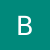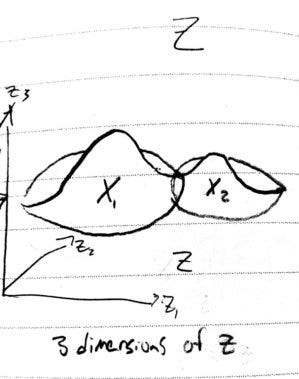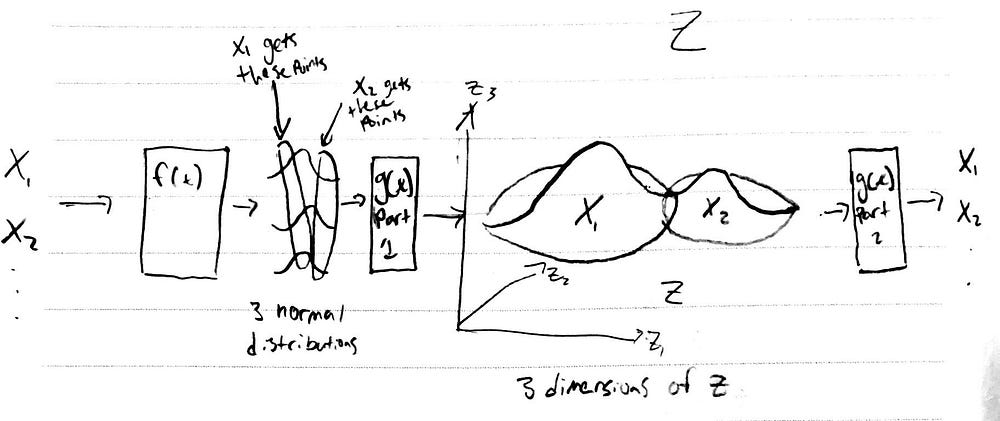Variational Autoencoder (VAE)

Source: Deep Learning on Medium

This directly builds off my previous post on Latent Variable Models found here: https://medium.com/datadriveninvestor/latent-variable-models-and-autoencoders-97c44858caa0 . If you haven’t read and are not familiar with the fundamentals of VAEs I recommend you go through it or at least look through the this tutorial on VAEs that I am following: https://arxiv.org/pdf/1606.05908.pdf.

Background

Desire — To generate new, similar, data from existing data.

In the last post we left with an idea of how we could structure our latent space to generate our desired output of images similar to our training examples. The figure below is what we came up with. We feed each training example through a function, f(X), which maps to a point in a latent space. Our output function, g(x), can be used to sample from around that point to generate our input or things similar to the input.

General Goal — To find how to form our encoder and decoder to generate and output as similar as possible to P(X) that we can sample from. We also decided we want to maximize the equality of the equation below:

To do this we need to find how to measure each term in the integral: P(X|z), and P(z). This turns out to be a little tricky.

Practically Implementing our Latent Variable Model

We start by going deep into how to find P(z) and the dimensions of z. Recalling the example of generating a face, z only had one dimension which consisted of two possible values that corresponded to outputting a male or female face. In practice, such a low dimensional latent space is not useful. We want our network to capture other features of a face, like maybe how round it is, or the hair length, or the eye to nose to mouth to pupil ratio. Ideally the model captures features that we don’t explicitly define but are optimally useful to recreate a convincing output. These are features and relationships between features that we would never come up with since they are so esoteric. Each feature exists on a separate dimension of z and we can say z has d dimensions. In our model, we carved out space in z to follow a normal distribution for each training example. Since we want to be able to sample anywhere in z it makes sense that we want to push each training example’s distribution to be as close as possible and even overlap (we do this with our optimization function). The overlap parts warp the distribution of z to no longer have clearly defined normal distributions scattered but to be distributed in a large oddly warped shape which we cannot predict.See how X1 and X2 modify each other so the space is warped in an unusual way

Where we could have easily encoded z before by stating a mean and variance for each training example we now have to determine how to encode the shape of z. It seems that VAEs use a property/trick of normal distributions to do this. We operate under the assumption that there is no easy way to interpret z of d dimensions but we can use d different variables that EACH follow a normal distribution mapped through a function to generate our space in z for our training example X (I wonder if each variable could follow any distribution…).The inputs are mapped in f(X) to d normal distributions which then are mapped, using g(z) to our desired latent space. This latent space is then used to reconstruct our inputs. I circle parts of 3 normal distributions to exemplify how points could be used to map to the latent space. A real model would have a much more complex mapping.

So, to make clear, the first picture in the article I showed is not exactly correct since the z space shown is actually generated as part of g(x) and our latent variables are d normal distributions which map to that space.

With this, we now have a way to encode our latent space, P(z), into d normal distributions. Again, the higher d is, the more features are passed from the encoder to the decoder.

We run into one more issue when implementing our latent variable model: that to generate X from anywhere around z|X is extremely unlikely so P(X) ends up having a very low variance and our distributions in z for each training example do not come close to each other. To address this we introduce a new distribution Q(z) which is some ideal distribution where all of our distributions in z are close to each other as we desire. I think of it as some kind of warping/folding of P(z) to now look how we want.

Given the goal of making P(z) similar to Q(z), we can now create a regularization to move them closer together. The popular regularization technique used to measure the difference between distributions is called KL-Divergence and follows the form below.

So we are finding the KL-Divergence between Q(z) and P(z|X). Note, the equation isn’t symmetric, so D[Q(z)||P(z|X)] != D[P(z|X)||Q(z)]. To interpret the right hand side consider that taking the log_2 of a number gives the number of bits it would take to store that number. This corresponds to the amount of information needed to encode that number. Essentially we are comparing information/probabilities at corresponding points in each distribution.

In the image above you can see how corresponding z values across the two distributions are unequal. With the KL-Divergence, we compare each corresponding point and compute the difference across the entire Q(z) area. It is intuitive to see this tells us how far one distribution is from the other and minimizing this term moves the distributions close to each other. Below I follow the math in the VAE Tutorial paper which pretty much just uses Bayes rule to add P(X) to the equation, a term we want to maximize.

We end up with:

Start with interpreting the left hand side. Our goal is to maximize log P(X). The degree we maximize it dictates the variance of the output of X. The KL-Divergence term entails minimizing the difference between our P and Q distributions given X. This should make sense since we designed Q to match P at z|X. The right hand side I think can be interpreted by minimizing the distance between our Q distribution and P given z. This gives the optimal space to generate X from. We then take an expectation over that space and see what the probability of generating X is from it. So essentially this looks like our optimal encoder decoder, and we can modify these divergence terms to modify the P(X|z).

Since we are getting P(z|X) to match Q(z|X) we can choose whatever distribution we want to match Q. Apparently the normal distribution, again, is a good option.

To optimize this function we now need to be able to determine the values of each distribution. After that we will need to determine the gradient of the equation and find how to optimize. There are a few steps/ “tricks” to do this, so I will save that for the next post.

Final Thoughts

I think a confusing point in understanding VAE’s is that the normal distribution is used in three different ways, not just one as a quick skim of a blog would lead us to believe. To recap, they are:

1. Attempting to have the probability of each training example when sampled from an area of the latent space equal the normal distribution
2. Generating the latent space distribution, P(z) of dimension d, using d normal distributions
3. Enforcing P(z) towards Q(z) by setting Q(z) to the normal distribution

With a fundamental understanding of the VAE, in the next post I want to finalize our understanding of the VAE. Following that, I will explore trading Q(z) for a different distribution called the Wasserstein Distance.